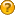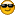###Author Topic: Texture doesn't seem to apply to a sphere  (Read 1605 times)

####lawless_c

• int
•• Posts: 95##### Texture doesn't seem to apply to a sphere
« on: January 06, 2014, 12:27:19 am »
I'm having what is likely a very minor issue applying a texture to a sphere. In the right of the image i have the texture/normalmap etc of portion of the earth working on a flat plane. However it seems to fail completely when i try apply to this to a sphere.Have i got something wrong here? e.g. texture size is 512x512?

####Lobby##### Re: Texture doesn't seem to apply to a sphere
« Reply #1 on: January 06, 2014, 12:50:15 pm »
Where do you have the sphere mesh from?
The mesh should have so called texture coordiantes which determine how texture is wrapped on it. If you used Primitives.getSphere() to get the sphere then I think you should use another method to get a sphere (even if you used Object3D.calcTextureWrapSpherical() on the sphere).

Here a little class to create a sphere with usable texture coords (use ExtendedPrimitives.createSphere()):
Code: [Select]
`public class ExtendedPrimitives { /** * Creates a sphere with a specific radius. * @param radius of the sphere * @param quads Number of quads that should be used for the sphere. You should seriously not use more quads than * you need! * @return the created sphere */ public static Object3D createSphere(float radius, int quads) { float size = 2 * radius; return createEllipsoid(new SimpleVector(size, size, size), quads); } /** * Creates an ellipsoid with given width, height and depth. * @param size Vector that contains width, height and depth * @param quads Number of quads that should be used for the sphere. You should seriously not use more quads than * you need! * @return the created ellipsoid */ public static Object3D createEllipsoid(SimpleVector size, int quads) { int yQuads = Math.max(quads / 2 + 1, 3); Object3D obj = new Object3D(2 * quads * yQuads); // Prepare vertices SimpleVector[][] v = new SimpleVector[quads][yQuads]; for (int y = 0; y < yQuads; y++) { float yAngle = (float) (Math.PI * y / (yQuads - 1)); float yPos = - 0.5f * size.y * (float) Math.cos(yAngle); float yRadius = (float) Math.sin(yAngle); for (int x = 0; x < quads; x++) { float xAngle = (float) (2 * Math.PI * x / quads); float xPos = 0.5f * size.x * (float) Math.cos(xAngle) * yRadius; float zPos = 0.5f * size.z * (float) Math.sin(xAngle) * yRadius; v[x][y] = new SimpleVector(xPos, yPos, zPos); } } // Create quads float v0 = 0; float v1 = 1f / (yQuads - 1); float vStep = v1; for (int y = 0; y + 1 < yQuads; y++) { for (int x = 0; x < quads; x++) { float u0 = 2f * (float) x / quads; float u1 = 2f * (float) (x + 1) / quads; if (y > 0) { obj.addTriangle(v[x][y], u0, v0, v[x][y + 1], u0, v1, v[(x + 1) % quads][y], u1, v0); } if (y + 2 < yQuads) { obj.addTriangle(v[(x + 1) % quads][y], u1, v0, v[x][y + 1], u0, v1, v[(x + 1) % quads][y + 1], u1, v1); } } v0 = v1; v1 += vStep; } return obj; }`
This methods, together with some others that also create primitives, will be included in jpct.util next time. So you could also wait until next release.
« Last Edit: January 06, 2014, 03:08:17 pm by Lobby »

####lawless_c

• int
•• Posts: 95##### Re: Texture doesn't seem to apply to a sphere
« Reply #2 on: January 06, 2014, 10:45:11 pm »
Amazing , it works perfectly!####Lobby##### Re: Texture doesn't seem to apply to a sphere
« Reply #3 on: January 06, 2014, 11:12:21 pm »
NiceYou may want that the texture isn't wrapped through the sphere two time - you can do this by using this method:
Code: [Select]
`/** * Creates an ellipsoid with given width, height and depth. * @param size Vector that contains width, height and depth * @param quads Number of quads that should be used for the sphere. You should seriously not use more quads than * you need! * @param uScale texture u scale, default is 2f * @param vScale texture v scale, default is 1f * @return the created ellipsoid */ public static Object3D createEllipsoid(SimpleVector size, int quads, float uScale, float vScale) { int yQuads = Math.max(quads / 2 + 1, 3); Object3D obj = new Object3D(2 * quads * yQuads); // Prepare vertices SimpleVector[][] v = new SimpleVector[quads][yQuads]; for (int y = 0; y < yQuads; y++) { float yAngle = (float) (Math.PI * y / (yQuads - 1)); float yPos = - 0.5f * size.y * (float) Math.cos(yAngle); float yRadius = (float) Math.sin(yAngle); for (int x = 0; x < quads; x++) { float xAngle = (float) (2 * Math.PI * x / quads); float xPos = 0.5f * size.x * (float) Math.cos(xAngle) * yRadius; float zPos = 0.5f * size.z * (float) Math.sin(xAngle) * yRadius; v[x][y] = new SimpleVector(xPos, yPos, zPos); } } // Create quads float v0 = 0; float v1 = vScale / (yQuads - 1); float vStep = v1; for (int y = 0; y + 1 < yQuads; y++) { for (int x = 0; x < quads; x++) { float u0 = uScale * (float) x / quads; float u1 = uScale * (float) (x + 1) / quads; if (y > 0) { obj.addTriangle(v[x][y], u0, v0, v[x][y + 1], u0, v1, v[(x + 1) % quads][y], u1, v0); } if (y + 2 < yQuads) { obj.addTriangle(v[(x + 1) % quads][y], u1, v0, v[x][y + 1], u0, v1, v[(x + 1) % quads][y + 1], u1, v1); } } v0 = v1; v1 += vStep; } return obj; }`
Now, create your sphere with something like createEllipsoid(new SimpleVector(1,1,1), 32, 1f, 1f) then I think it is perfect####lawless_c

• int
•• Posts: 95##### Re: Texture doesn't seem to apply to a sphere
« Reply #4 on: January 08, 2014, 01:08:56 am »
Does it get wrapped twice? i'll try the ellipsoid then.

In the meantime i've added a cloudsphere####lawless_c

• int
•• Posts: 95##### Re: Texture doesn't seem to apply to a sphere
« Reply #5 on: January 09, 2014, 01:03:56 am »
I understand now, there was some annoying distortions that was particularly noticeable when looking at Africa which was very elongated. When i switched to the the ellipsoid that fixed it!Thanks